﻿ EcoAluminum spacer　/　CL-BE　【Hirosugi-Keiki Co.,Ltd.】

#### Low-Pb aluminum spacer( Hollow tube) Black anodized　/ CL-BE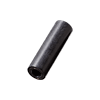Material：Lead-free free-cut aluminum(CB156)Lead content ≤10 ppm (forM2) Lead-free free-cut aluminum(KS26)Lead content ≤300 ppm (for M2.6) Treatment：Black alumite(BAL)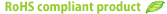■CL-2000BE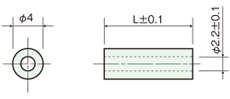MOQ:50pcs

Screw diameter

L Product Code Unit Price(\$)
(For M=2) 1 CL-2001BE 0.42
1.5 　CL-2001.5BE 0.43
2 CL-2002BE 0.43
2.5 　CL-2002.5BE 0.43
3 CL-2003BE 0.43
3.5 　CL-2003.5BE 0.43
4 CL-2004BE 0.43
4.5 　CL-2004.5BE 0.44
5 CL-2005BE 0.44
5.5 　CL-2005.5BE 0.44
6 CL-2006BE 0.44
6.5 　CL-2006.5BE 0.44
7 CL-2007BE 0.44
7.5 　CL-2007.5BE 0.44
8 CL-2008BE 0.44
8.5 　CL-2008.5BE 0.46
9 CL-2009BE 0.46
9.5 　CL-2009.5BE 0.46
10 CL-2010BE 0.46
11 CL-2011BE 0.46
12 CL-2012BE 0.47
13 CL-2013BE 0.47
14 CL-2014BE 0.48
15 CL-2015BE 0.48
16 CL-2016BE 0.48
17 CL-2017BE 0.49
18 CL-2018BE 0.49
19 CL-2019BE 0.51
20 CL-2020BE 0.56
■CL-2600BE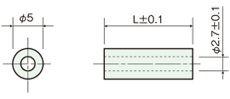MOQ:50pcs

Screw diameter

L Product Code Unit Price(\$)
(For M=2.6) 1 CL-2601BE 0.43
1.5 　CL-2601.5BE 0.45
2 CL-2602BE 0.45
2.5 　CL-2602.5BE 0.44
3 CL-2603BE 0.44
3.5 　CL-2603.5BE 0.44
4 CL-2604BE 0.44
4.5 　CL-2604.5BE 0.45
5 CL-2605BE 0.45
5.5 　CL-2605.5BE 0.45
6 CL-2606BE 0.45
6.5 　CL-2606.5BE 0.46
7 CL-2607BE 0.45
7.5 　CL-2607.5BE 0.46
8 CL-2608BE 0.46
8.5 　CL-2608.5BE 0.46
9 CL-2609BE 0.46
9.5 　CL-2609.5BE 0.46
10 CL-2610BE 0.46
11 CL-2611BE 0.48
12 CL-2612BE 0.48
13 CL-2613BE 0.48
14 CL-2614BE 0.49
15 CL-2615BE 0.49
16 CL-2616BE 0.49
17 CL-2617BE 0.51
18 CL-2618BE 0.51
19 CL-2619BE 0.53
20 CL-2620BE 0.61
■CL-300BE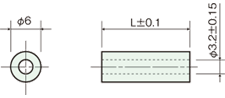Slot marked on the cut end surface
MOQ:50pcs

Screw diameter

L Product code Unit Price(\$)
(For M=3) 1 CL-301BE 0.44
1.5 CL-301.5BE 0.46
2 CL-302BE 0.46
2.5 CL-302.5BE 0.46
3 CL-303BE 0.46
3.5 CL-303.5BE 0.46
4 CL-304BE 0.46
4.5 CL-304.5BE 0.47
5 CL-305BE 0.47
5.5 CL-305.5BE 0.47
6 CL-306BE 0.47
6.5 CL-306.5BE 0.47
7 CL-307BE 0.47
7.5 CL-307.5BE 0.47
8 CL-308BE 0.47
8.5 CL-308.5BE 0.48
9 CL-309BE 0.48
9.5 CL-309.5BE 0.48
10 CL-310BE 0.48
11 CL-311BE 0.49
12 CL-312BE 0.49
13 CL-313BE 0.50
14 CL-314BE 0.50
15 CL-315BE 0.50
16 CL-316BE 0.50
17 CL-317BE 0.52
18 CL-318BE 0.52
19 CL-319BE 0.54
20 CL-320BE 0.62
21 CL-321BE 0.84
22 CL-322BE 0.84
23 CL-323BE 0.84
24 CL-324BE 0.84
25 CL-325BE 0.84
26 CL-326BE 0.84
27 CL-327BE 0.84
28 CL-328BE 0.84
29 CL-329BE 0.84
30 CL-330BE 1.01
35 CL-335BE 1.07
40 CL-340BE 1.12
45 CL-345BE 1.34
50 CL-350BE 1.40
■CL-400BE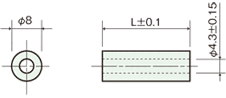Slot marked on the cut end surface
MOQ:50pcs

Screw diameter

L Product code Unit Price(\$)
(For M=4) 1 CL-401BE 0.47
1.5 CL-401.5BE 0.47
2 CL-402BE 0.47
2.5 CL-402.5BE 0.47
3 CL-403BE 0.47
3.5 CL-403.5BE 0.48
4 CL-404BE 0.48
4.5 CL-404.5BE 0.48
5 CL-405BE 0.48
5.5 CL-405.5BE 0.49
6 CL-406BE 0.49
6.5 CL-406.5BE 0.49
7 CL-407BE 0.49
7.5 CL-407.5BE 0.49
8 CL-408BE 0.49
8.5 CL-408.5BE 0.49
9 CL-409BE 0.50
9.5 CL-409.5BE 0.50
10 CL-410BE 0.50
11 CL-411BE 0.51
12 CL-412BE 0.51
13 CL-413BE 0.52
14 CL-414BE 0.53
15 CL-415BE 0.54
16 CL-416BE 0.56
17 CL-417BE 0.56
18 CL-418BE 0.57
19 CL-419BE 0.58
20 CL-420BE 0.66
21 CL-421BE 0.88
22 CL-422BE 0.88
23 CL-423BE 0.88
24 CL-424BE 0.88
25 CL-425BE 0.88
26 CL-426BE 0.88
27 CL-427BE 0.88
28 CL-428BE 0.88
29 CL-429BE 0.88
30 CL-430BE 1.04
35 CL-435BE 1.10
40 CL-440BE 1.16
45 CL-445BE 1.38
50 CL-450BE 1.43
■CL-500BE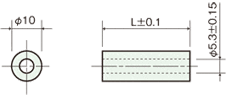Slot marked on the cut end surface
MOQ:50pcs

Screw diameter

L Product code Unit Price(\$)
(For M=5) 1 CL-501BE 0.49
1.5 CL-501.5BE 0.49
2 CL-502BE 0.49
2.5 CL-502.5BE 0.49
3 CL-503BE 0.49
3.5 CL-503.5BE 0.50
4 CL-504BE 0.50
4.5 CL-504.5BE 0.50
5 CL-505BE 0.50
5.5 CL-505.5BE 0.51
6 CL-506BE 0.51
6.5 CL-506.5BE 0.51
7 CL-507BE 0.51
7.5 CL-507.5BE 0.52
8 CL-508BE 0.52
8.5 CL-508.5BE 0.53
9 CL-509BE 0.53
9.5 CL-509.5BE 0.54
10 CL-510BE 0.54
11 CL-511BE 0.56
12 CL-512BE 0.57
13 CL-513BE 0.57
14 CL-514BE 0.58
15 CL-515BE 0.59
16 CL-516BE 0.60
17 CL-517BE 0.61
18 CL-518BE 0.62
19 CL-519BE 0.63
20 CL-520BE 0.73
21 CL-521BE 0.90
22 CL-522BE 0.90
23 CL-523BE 0.90
24 CL-524BE 0.90
25 CL-525BE 0.90
26 CL-526BE 0.90
27 CL-527BE 0.90
28 CL-528BE 0.90
29 CL-529BE 0.90
30 CL-530BE 1.07
35 CL-535BE 1.12
40 CL-540BE 1.18
45 CL-545BE 1.40
50 CL-550BE 1.46
■CL-600BE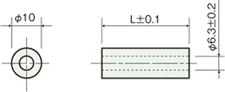Length less than 20 mm. slot marked on the cut end surface
MOQ:50pcs

Screw diameter

L Product code Unit Price(\$)
(For M=6) 1 CL-601BE 0.56
2 CL-602BE 0.57
3 CL-603BE 0.57
4 CL-604BE 0.58
5 CL-605BE 0.59
6 CL-606BE 0.60
7 CL-607BE 0.61
8 CL-608BE 0.61
9 CL-609BE 0.62
10 CL-610BE 0.63
11 CL-611BE 0.64
12 CL-612BE 0.66
13 CL-613BE 0.67
14 CL-614BE 0.67
15 CL-615BE 0.68
16 CL-616BE 0.69
17 CL-617BE 0.70
18 CL-618BE 0.71
19 CL-619BE 0.72
20 CL-620BE 0.73
22 CL-622BE 0.90
25 CL-625BE 0.92
28 CL-628BE 1.09
30 CL-630BE 1.26
35 CL-635BE 1.31
40 CL-640BE 1.37
45 CL-645BE 1.42
50 CL-650BE 1.48